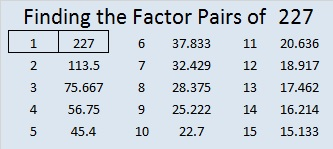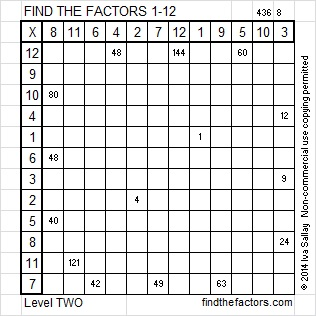# 227 and Level 2

• 227 is a prime number.
• Prime factorization: 227 is prime.
• The exponent of prime number 227 is 1. Adding 1 to that exponent we get (1 + 1) = 2. Therefore 227 has exactly 2 factors.
• Factors of 227: 1, 227
• Factor pairs: 227 = 1 x 227
• 227 has no square factors so its square root cannot be simplified. √227 ≈ 15.067How do we know that 227 is a prime number? If 227 were not a prime number, then it would be divisible by at least one prime number less than or equal to √227 ≈ 15.067. Since 227 cannot be divided evenly by 2, 3, 5, 7, 11, or 13, we know that 227 is a prime number.Excel file of puzzles and previous week’s factor solutions: 12 Factors 2014-09-08This site uses Akismet to reduce spam. Learn how your comment data is processed.